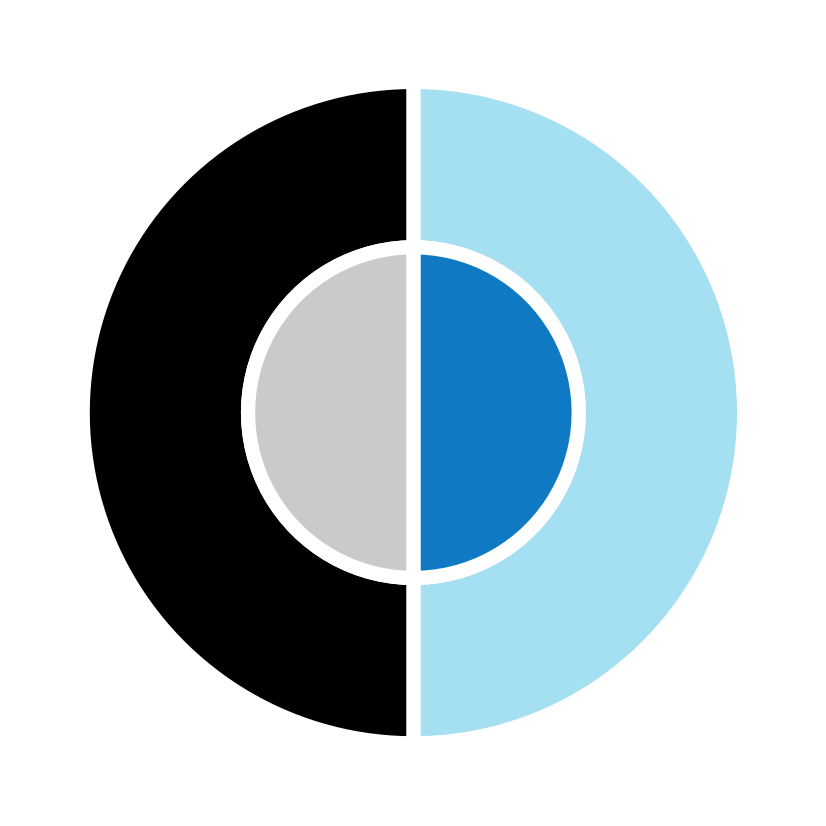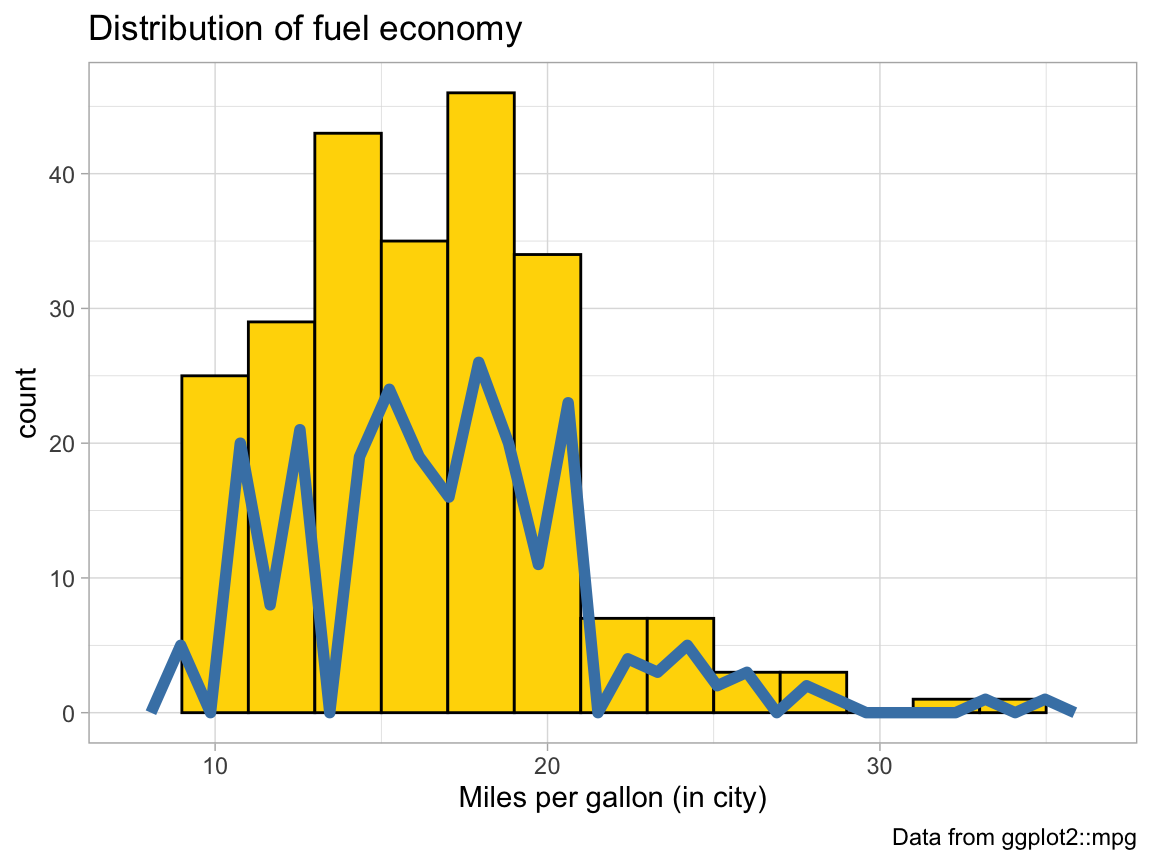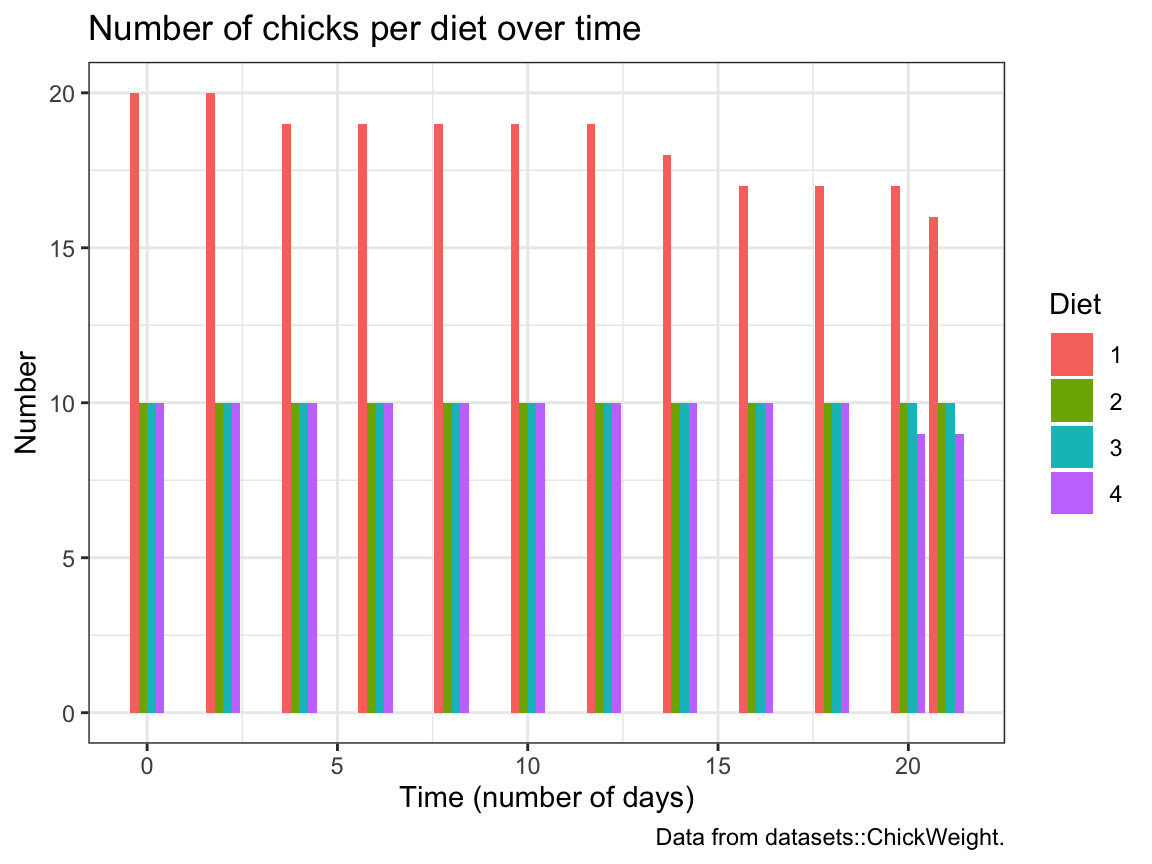## 2.7 ExercisesThe following exercises allow you to apply the ggplot() commands introduced in this chapter.

### 2.7.1 Exercise 1

#### Scattered highways

A scatterplot shows a data point (observation) as a function of 2 (typically continuous) variables x and y. This allows judging the relationship between x and y in the data.

1. Use the mpg data of ggplot2 to create a scatterplot that shows a car’s fuel economy on the highway (on the y-axis) as a function of its fuel economy in the city (on the x-axis). How would you describe this relationship?

2. Does your plot suffer from overplotting? If so, create at least 2 different versions that address this problem.

3. Add informative titles, labels, and a theme to the plot.

4. Group the points in your scatterplot by the class of vehicles (in at least 2 different ways).

### 2.7.2 Exercise 2

#### Strange histograms

The following plot repeats the histogram code from above (to plot the distribution of fuel economy in city environments), but adds a frequency polygon as a 2nd geom (see ?geom_freqpoly).

# Plot from above with an additional geom:
ggplot(mpg, aes(x = cty)) +    # set mappings for ALL geoms
geom_histogram(aes(x = cty), binwidth = 2, fill = "gold", color = "black") +
geom_freqpoly(color = "steelblue", size = 2) +
labs(title = "Distribution of fuel economy",
x = "Miles per gallon (in city)",
caption = "Data from ggplot2::mpg") +
theme_light()1. Why is the (blue) line of the polygon lower than the (yellow) bars of the histogram?

2. Change 1 value in the code so that both (lines and bars) have the same heights.

3. The code above repeats the aesthetic mapping aes(x = cty) in 2 locations. Which of these can be deleted without changing the resulting graph? Why?

4. Why can’t we simply replace geom_freqpoly by geom_line or geom_smooth to get a similar line?

### 2.7.3 Exercise 3

#### Cylinder bars

Let’s create some bar plots with the ggplot2::mpg data.

1. Plot the number or frequency of cases by cyl as a bar plot (in at least 2 different ways).

2. Plot the proportion of cases in the mpg data by cyl (in at least 2 different ways).

3. Create a better and prettier version by adding different colors, appropriate labels, and a suitable theme to your plot.

See Appendix D for additional color options in R.

### 2.7.4 Exercise 4

#### Chick diets

The ChickWeight data (contained in the datasets package of R) contains the results of an experiment that measures the effects of Diet on the early growth of chicks.

1. Save the ChickWeight data as a tibble cw and inspect its dimensions and variables.
# ?datasets::ChickWeight

# (a) Save data as tibble and inspect:
cw <- as_tibble(ChickWeight)
# cw  # 578 observations (rows) x 4 variables (columns)
1. Create a line plot showing the weight development of each indivdual chick (on the y-axis) over Time (on the x-axis) for each Diet (in 4 different facets).

2. The following bar chart shows the number of chicks per Diet over Time.
We see that the initial Diet groups contain a different numbers of chicks and some chicks drop out over Time:Try re-creating this plot (with geom_bar and dodged bar positions).

### 2.7.5 Exercise 5

#### Participant plots

Use the p_info data from Exercise 6 of Chapter 1 (available as posPsy_p_info in the ds4psy package) to create some plots that descripte the sample of participants:

# Load data:
p_info <- ds4psy::posPsy_p_info  # from ds4psy package
# all.equal(p_info, p_info_2)

# dim(p_info)      # 295 rows, 6 columns
# p_info           # prints a summary of the table/tibble
# glimpse(p_info)  # shows the first values for 6 variables (columns)

# Turn some categorial values into factors:
p_info$sex <- as.factor(p_info$sex)
p_info$intervention <- as.factor(p_info$intervention)

# p_info  # Note that the variables intervention and sex are now listed as <fct>.
1. A histogram that shows the distribution of participant age in 3 ways:
• overall,
• separately for each sex, and
• separately for each intervention.
1. A bar plot that
• shows how many participants took part in each intervention; or
• shows how many participants of each sex took part in each intervention.

### 2.7.6 Exercise 6

#### Visual illusions

Not all visualizations need to depict data. For instance, visual illusions reveal something about the mechanisms of our visual system.

1. Look up the term grid illusion (e.g., on Wikipedia) and re-create the so-called Hermann grid illusion using ggplot2.

Hints:

• We can call ggplot() without any data and aesthetics arguments and then explicitly provide the positions of desired lines (in geom_hline() and geom_vline() commands).

• Creating a dark background in ggplot2 requires a combination of theme, plot.background and panel.background commands. A decent compromise is using theme_dark().

1. Making visual illusions disappear: Adjust the alpha parameter of the (horizontal and vertical) grid lines until you have the impression that the dark dots disappear.

2. Use the function make_grid() (with two options x and y) to create a data object dots.

# Make a nx-by-ny grid of x-y coordinates:
make_grid <- function(x = 5, y = 5){

xs <- -x:x
ys <- -y:y

dots <- tibble::tibble(x = rep(xs, times = length(ys)),
y = rep(ys, each = length(xs)))

return(dots)
}

Then use dots as data input to a ggplot() call to create a Scintillating grid illusion.

Hint: The code is almost identical to the Hermann grid illusion above, but we need to add geom_point() to create x by y points. The dots dataset contains the coordinates of these points.

This concludes our first set of exercises on visualizing data — but ggplot2 will still feature prominently in the following chapters of this book.